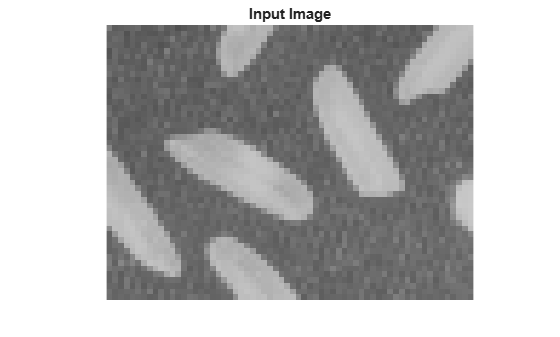# visionhdl.ImageStatistics

Mean, variance, and standard deviation

## Description

`visionhdl.ImageStatistics` calculates the mean, variance, and standard deviation of streaming video data. Each calculation is performed over all pixels in the input region of interest (ROI). The object implements the calculations using hardware-efficient algorithms.

This object uses a streaming pixel interface with a structure for frame control signals. This interface enables the object to operate independently of image size and format and connect with other Vision HDL Toolbox™ objects. The object accepts pixel data as integer, fixed-point, or floating-point data types. The object accepts control signals as a structure containing five signals. The control signals indicate the validity of each pixel and its location in the frame. To convert a pixel matrix into a pixel stream and control signals, use the `visionhdl.FrameToPixels` object. For a full description of the interface, see Streaming Pixel Interface.

• To change the size and dimensions of the ROI, you can manipulate the input video stream control signals. See Regions of Interest.

• The number of valid pixels in the input image affect the accuracy of the mean approximation. To avoid approximation error, use an image that contains fewer than 64 pixels, a multiple of 64 pixels up to 642 pixels, a multiple of 4096 pixels up to 643 pixels, or a multiple of 643 pixels up to 644 pixels. For details of the mean approximation, see Algorithm.

• The object calculates statistics over frames up to 644 (16,777,216) pixels in size.

Note

Starting in R2016b, instead of using the `step` method to perform the operation defined by the System object™, you can call the object with arguments, as if it were a function. For example, `y = step(obj,x)` and `y = obj(x)` perform equivalent operations.

## Construction

`S = visionhdl.ImageStatistics` returns a System object, `S`, that calculates the mean, variance, and standard deviation of each frame of a video stream.

`S = visionhdl.ImageStatistics(Name,Value)` returns a System object, `S`, with additional options specified by one or more `Name,Value` pair arguments. `Name` is a property name and `Value` is the corresponding value. `Name` must appear inside single quotes (`''`). You can specify several name-value pair arguments in any order as `Name1,Value1,...,NameN,ValueN`. Properties not specified retain their default values.

## Properties

 `mean` Calculate the mean of each input frame. If you set this property to `false`, the `step` method does not return this output. Default: `true` `variance` Calculate the variance of each input frame. If you set this property to `false`, the `step` method does not return this output. Default: `true` `stdDev` Calculate the standard deviation of each input frame. If you set this property to `false`, the `step` method does not return this output. Default: `true`

## Methods

 step Calculate the contribution of one pixel to the mean, variance, and standard deviation of a video stream
Common to All System Objects
`release`

Allow System object property value changes

## Examples

collapse all

This example computes the mean, variance, and standard deviation of a thumbnail image.

Load the source image from a file. Select a portion of the image matching the desired test size.

```frmOrig = imread('rice.png'); frmActivePixels = 64; frmActiveLines = 48; frmInput = frmOrig(1:frmActiveLines,1:frmActivePixels); figure imshow(frmInput,'InitialMagnification',300) title 'Input Image'```Create a serializer object and define inactive pixel regions.

```frm2pix = visionhdl.FrameToPixels(... 'NumComponents',1,... 'VideoFormat','custom',... 'ActivePixelsPerLine',frmActivePixels,... 'ActiveVideoLines',frmActiveLines,... 'TotalPixelsPerLine',frmActivePixels+10,... 'TotalVideoLines',frmActiveLines+10,... 'StartingActiveLine',6,... 'FrontPorch',5);```

Create an object that returns mean, variance, and standard deviation.

` stats = visionhdl.ImageStatistics();`

Serialize the test image by calling the serializer object. `pixIn` is a vector of intensity values. `ctrlIn` is a vector of control signal structures.

Note: This object syntax runs only in R2016b or later. If you are using an earlier release, replace each call of an object with the equivalent `step` syntax. For example, replace `myObject(x)` with `step(myObject,x)`.

`[pixIn,ctrlIn] = frm2pix(frmInput);`

Prepare to process pixels by preallocating output vectors.

```[~,~,numPixelsPerFrame] = getparamfromfrm2pix(frm2pix); validOut = false(numPixelsPerFrame,1); mean = zeros(numPixelsPerFrame,1,'uint8'); variance = zeros(numPixelsPerFrame,1,'uint8'); stddev = zeros(numPixelsPerFrame,1,'uint8');```

For each pixel in the stream, increment the internal statistics.

```for p = 1:numPixelsPerFrame [mean(p),variance(p),stddev(p),validOut(p)] = stats(pixIn(p),ctrlIn(p)); end```

The results are valid when `validOut` is returned `true`.

`mean = mean(validOut==1)`
```mean = uint8 125 ```
`variance = variance(validOut==1)`
```variance = uint8 255 ```
`stddev = stddev(validOut==1)`
```stddev = uint8 36 ```

## Algorithms

This object implements the algorithms described on the Image Statistics block reference page.

| | (Computer Vision Toolbox) | (Computer Vision Toolbox) | (Computer Vision Toolbox) | (Image Processing Toolbox) | (Image Processing Toolbox)

Introduced in R2015a

## SupportGet trial now